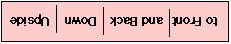Serial Bit Order

If one looks at the waveform for either an asynchronous or a synchronous stream of bytes (characters) using an oscilloscope, then one notices that things are not quite as one expects. There are two things which may be unexpected:

• Since line drivers invert the signal prior to transmission (and line receivers do an inversion on reception), the signal that appears on the wires is usually upside down. That is a "1" or "idle" period is represented by a low voltage and a "0" or "start bit" is represented as a high voltage.
• Ever since the start of asynchronous communications, the bits have been sent down the wire with the least significant data bit first and the most significant bit last. All that this really implies is a change in order of the lines connected from the computer's data bus to the transmit and receive shift registers.

So, what one sees on the oscilloscope is:Asynchronous Transmission

For an asynchronous transmission, this means that the line usually is at the low voltage (OV or -12V) and that a logic 0 is sent as a high voltage (+5V or +12V). The least significant bit of the character follows the start bit (rather than the most significant as one may have expected), followed by the rest of the bits in the byte, the parity bit (if present) and the stop bit.

Exception to the Rule

The bit order for all bits follows the above rules, with only one exception - that CRC appended to the end of some link layer frames.

Gorry Fairhurst - Date: 01/01/2001 EG3557